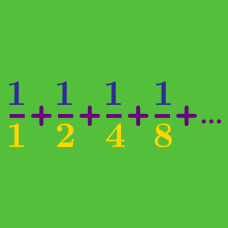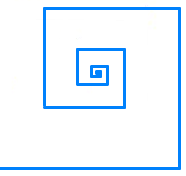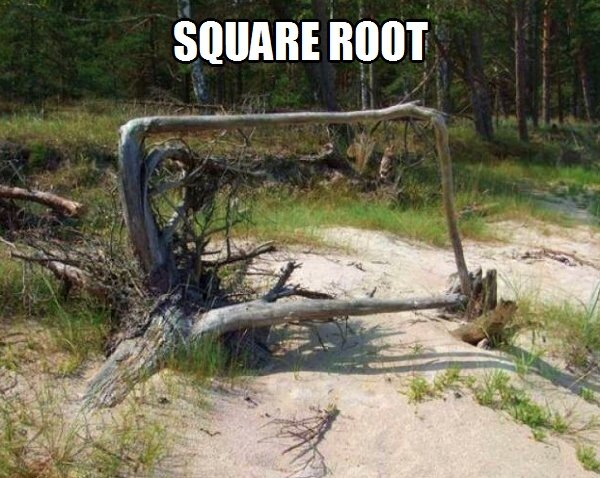Calculus

# Sequences and Series: Level 3 Challenges

If $$x^3, x, x^2$$ form an arithmetic progression (in the given order), find the sum of all possible values of $$x$$.

Clarification: An arithmetic progression is allowed to be a constant sequence.

In the answer options, $$\phi = \frac{ 1 + \sqrt{5} } { 2}$$ (the golden ratio).Cody has started running in a well-organized manner. He runs $$100 \text{ m}$$ east, then turns left and runs another $$10 \text{ m}$$ north, turns left and runs $$1 \text{ m},$$ again turns left and runs $$0.1 \text{ m},$$ and on the next turn $$0.01 \text{ m},$$ and so on.

Assuming that Cody can run in this pattern infinitely, the displacement from his initial position can be written as $$\frac{a}{\sqrt{b}}$$ with $$a$$ and $$b$$ being positive integers and $$b$$ square-free.

What is the value of $$a \times b?$$

\begin{align*} 10^{-1}(1 + 0.1 + 0.01& + 0.001 + \cdots) + \\ 10^{-2}(2 + 0.2 + 0.02& + 0.002 + \cdots) + \\ 10^{-3}(3 + 0.3 + 0.03& + 0.003 + \cdots) + \\ &. \\ &. \\ &. \\ \end{align*}

The above sum can be represented as $$\left(\dfrac{a}{b}\right)^{2}$$, where $$a$$ and $$b$$ are coprime positive integers. Find the value of $$a+b$$.

Clarification: In the $$n^\text{th}$$ term, after the string of $$n - 1$$ zeroes, the number under the bar is $$n$$.

If the sum of an arithmetic progression of six positive integer terms is 78, what is the greatest possible difference between consecutive terms?$\Large \sqrt{2 \sqrt{4 \sqrt{8 \sqrt{16\ldots}}} } = \, ?$

×

Problem Loading...

Note Loading...

Set Loading...